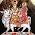VHDL coding tips and tricks: How to write the current simulation time to a file in VHDL

## Saturday, August 17, 2013

### How to write the current simulation time to a file in VHDL

In the past I have written few posts about file reading and writing in VHDL. In this post, we will address a specific issue related with testbench i.e writing the current simulation time in to a file. This kind of information is some times very useful for testing and debugging your design.

This can be done using the textio package in vhdl. Let me show an example.

library ieee;
use STD.textio.all;
use IEEE.STD_LOGIC_TEXTIO.all;

entity rand_gen is
end rand_gen;

architecture behavior of rand_gen is

begin

process
variable L: line;
variable T: time;
variable line_var : line;
file text_var : text;
begin
file_open(text_var,"time_file.txt",write_mode);  --open the file for writing.
for i in 1 to 10 loop
write(line_var, string'("The current simulation time is :"));
write(line_var, time'IMAGE(now));
writeline(text_var,line_var);
wait for 10000 ns;
end loop;
file_close(text_var);
wait;
end process;

end behavior;

After the above code is run for sufficient time, a file named time_file.txt will be created with the following contents:

The current simulation time is :0 ps
The current simulation time is :10000000 ps
The current simulation time is :20000000 ps
The current simulation time is :30000000 ps
The current simulation time is :40000000 ps
The current simulation time is :50000000 ps
The current simulation time is :60000000 ps
The current simulation time is :70000000 ps
The current simulation time is :80000000 ps
The current simulation time is :90000000 ps

The code simply runs a for loop for 10 times, with a delay of 10000 ns between each write operation.

The predefined function now returns the current simulation time. time'image(X) returns a string representation of X that is of type time.

The present simulation time is normally needed, when we need to know at what time exactly a certain event happens in vhdl. A well written testbench can make the testing process much more easier and fun.

Note :- The code was tested using Xilinx ISE 13.1. But it should work with other simulation tools as well.

#### 1 comment:

1.HI, help me out on this
Main memory is divided into 256 pages , each pages is 8 bits wide.
the module is called as REGISTER
Inputs are " DATA_BUS {7:0], REG_ADDRESS[7:0], RESET, CLK and Output is REG_DATA, How to initialise the module of the register whis is 256 pages and 8 bits wide, help me out on this